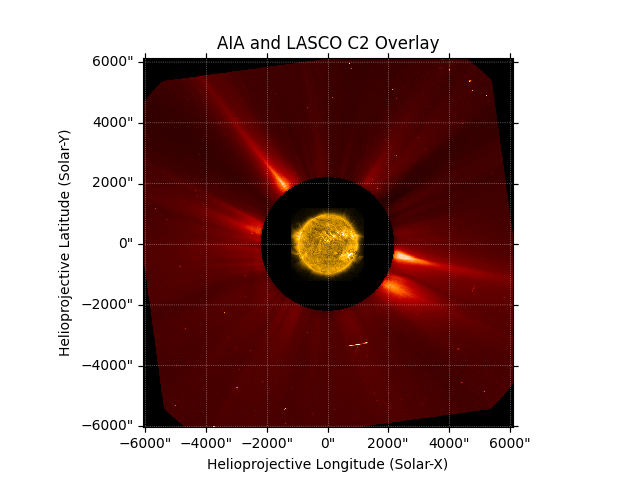# Overlay an AIA image on a LASCO C2 coronagraph#

This example shows the steps needed to overlay the disc and off-limb components of an AIA image within the masked occulter of a LASCO C2 image.

from datetime import datetime

import hvpy
import matplotlib.pyplot as plt
from hvpy.datasource import DataSource

import astropy.units as u
from astropy.coordinates import SkyCoord

import sunpy.data.sample
from sunpy.coordinates import Helioprojective
from sunpy.map import Map


First, we will acquire a calibrated LASCO C2 image from Helioviewer and create a map. hvpy uses the standard datetime instead of astropy.time.

lasco_jp2_file = hvpy.save_file(hvpy.getJP2Image(datetime(2011, 6, 7, 6, 34),
DataSource.LASCO_C2.value),
lasco_map = Map(lasco_jp2_file)
aia_map = Map(sunpy.data.sample.AIA_171_IMAGE)


In order to plot off-limb features of the AIA image.

Note that off-disk AIA data are not retained by default because an additional assumption is required to define the location of the AIA emission in 3D space. We can use assume_spherical_screen() to retain the off-disk AIA data. See Reprojecting Using a Spherical Screen for more reference.

projected_coord = SkyCoord(0*u.arcsec, 0*u.arcsec,
obstime=lasco_map.observer_coordinate.obstime,
frame='helioprojective',
observer=lasco_map.observer_coordinate,
rsun=aia_map.coordinate_frame.rsun)
projected_coord,
scale=u.Quantity(aia_map.scale),
instrument=aia_map.instrument,
wavelength=aia_map.wavelength)
# We use assume_spherical_screen to ensure that the off limb AIA pixels are reprojected
# otherwise it will only be the on disk pixels that are reprojected.
with Helioprojective.assume_spherical_screen(aia_map.observer_coordinate):


Finally, we plot the images by layering the AIA image on top of the LASCO C2 image.

fig = plt.figure()INFO: Missing metadata for solar radius: assuming the standard radius of the photosphere. [sunpy.map.mapbase]